# ACT Math - Trigonometry: Help and Review Chapter Exam

Exam Instructions:

Choose your answers to the questions and click 'Next' to see the next set of questions. You can skip questions if you would like and come back to them later with the yellow "Go To First Skipped Question" button. When you have completed the practice exam, a green submit button will appear. Click it to see your results. Good luck!

### Page 1

#### Question 2 2. Solve the following trigonometric equation for θ.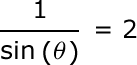#### Question 4 4. What's the value of sec2 (θ) given that the equation below is true?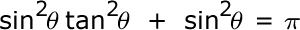### Page 2

#### Question 7 7. Solve for the value of x in the trigonometric equation below. (Use the domain -π/2 to π/2)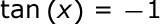#### Question 8 8. What is x?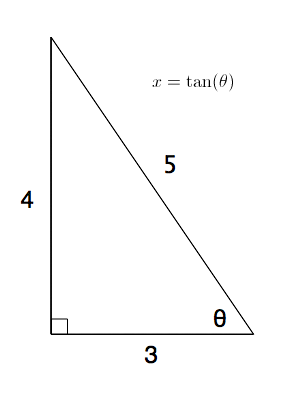#### Question 9 9. What is z in terms of x?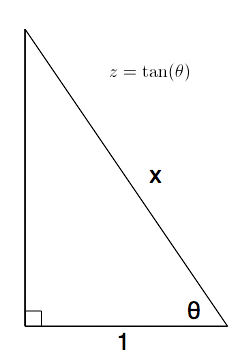#### Question 10 10. Solve the following equation for x.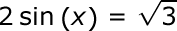### Page 3

#### Question 11 11. What is x?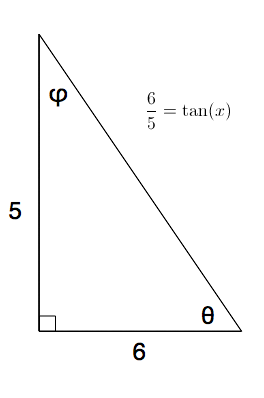#### Question 12 12. What is x?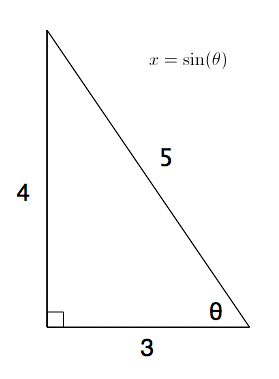#### Question 13 13. What is x?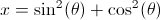#### Question 14 14. Solve the following trigonometric equation for x.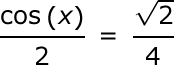### Page 4

#### Question 17 17. The lines a and b are parallel lines of length 5 and 2. The lines d and e are parallel to one another, and both are perpendicular to a and b. What is the length of c?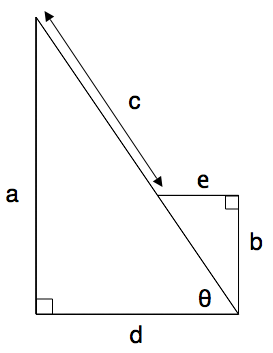#### Question 19 19. What's the value of 2sin(θ)cos(θ) given that the equation below is true?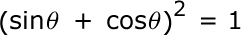#### Question 20 20. What is z in terms of x and y?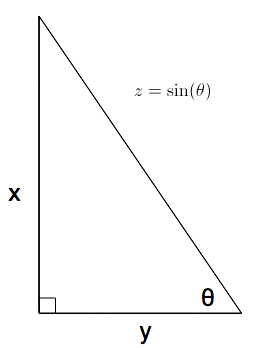### Page 5

#### Question 21 21. Perform the following calculation. Report the answer in radians.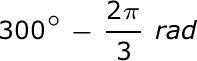#### Question 22 22. Simplify the following expression.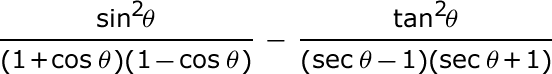#### Question 24 24. What is the following radian measure in degrees?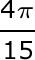#### Question 25 25. Solve the following equation for θ.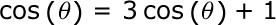### Page 6

#### Question 26 26. Simplify the following expression.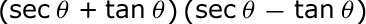#### Question 28 28. Convert the following measurement in radian to degrees.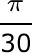#### Question 29 29. What is x?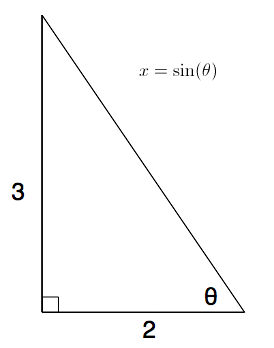#### ACT Math - Trigonometry: Help and Review Chapter Exam Instructions

Choose your answers to the questions and click 'Next' to see the next set of questions. You can skip questions if you would like and come back to them later with the yellow "Go To First Skipped Question" button. When you have completed the practice exam, a green submit button will appear. Click it to see your results. Good luck!

Support